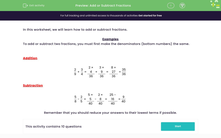### Comprehensive & curriculum aligned

In this worksheet, students will add and subtract fractions.Key stage:  KS 3

Curriculum topic:   Number

Curriculum subtopic:   Use Four Operations for All Numbers

Popular topics:   Fractions worksheets, Percentages worksheets

Difficulty level:#### Worksheet Overview

In this activity, we will learn how to add and subtract fractions.

Examples

To add or subtract two fractions, you must first make the denominators (bottom numbers) the same.

To do this, we need to find the lowest common multiple of the denominators.

In the example below, we have 9 and 4 as the denominators and the lowest common multiple of 9 and 4 is 36.

Next, we need to recognise what we did to each denominator to turn it into 36, and do the same thing to the numerator.

With 2/9, we multiplied the 9 by 4 to get 36, so we must multiply the 2 by 4 too. This gives us 8/36.

With 3/4, we multiplied the 4 by 9 to get 36, so we must multiply the 3 by 9 too. This gives us 27/36.

Now we can add the two fractions: 8/36 + 27/36 = 35/36.

We can't simplify this any further, so that is our answer.

 2 9
+
 3 4
=
 2 × 4 36
+
 3 × 9 36
=
 8 + 27 36
=
 35 36

In the example below, we find that 40 is the lowest common multiple of 8 and 5.

We multiplied 8 by 5 to get 40, so we multiply 5 by 5 to give us 25/40.

We multiplied 5 by 8 to get 40, so we multiply 2 by 8 to give us 16/40.

Finally, we can do our subtraction: 25/40 - 16/40 = 9/40.

We can't simplify this any further, so that is our answer.

 5 8
-
 2 5
=
 5 × 5 40
-
 2 × 8 40
=
 25 - 16 40
=
 9 40

Remember that you should always reduce your answers to their lowest terms if possible.

### What is EdPlace?

We're your National Curriculum aligned online education content provider helping each child succeed in English, maths and science from year 1 to GCSE. With an EdPlace account you’ll be able to track and measure progress, helping each child achieve their best. We build confidence and attainment by personalising each child’s learning at a level that suits them.

Get started